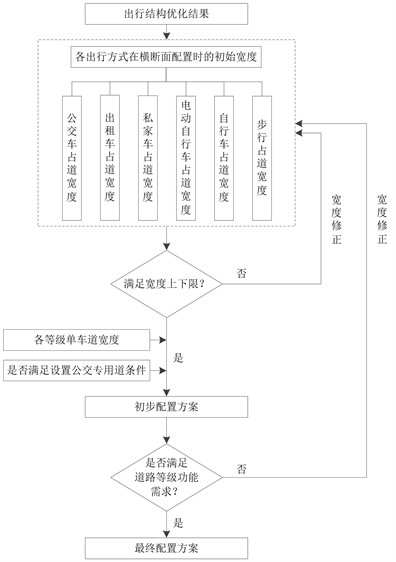# 基于合理出行结构的道路资源配置方法Road Resource Allocation Method Based on Reasonable Travel Structure

DOI: 10.12677/OJTT.2020.93017, PDF, HTML, XML, 下载: 10  浏览: 41

Abstract: With the improvement of living standards, the demand for resident travel is increasing, and the contradiction between supply and demand of urban roads gradually appears. Due to the lack of foresight in road planning and design in some cities, the road resources in built-up areas are li-mited and its allocation is irrational, resulting in frequent traffic congestion in recent years. By analyzing the relationship between the travel structure and the road resource allocation, the method of the road resource allocation based on a reasonable travel structure was proposed. And the feasibility of it was verified by applying it to Guilin.

1. 引言

2. 道路资源优化配置方法

2.1. 出行结构与道路资源关系分析

2.2. 基于合理出行结构的道路资源优化配置方法

2.2.1. 出行结构优化模型构建

1). 模型假设

2). 模型构建

$\left\{\begin{array}{l}\mathrm{max}P={\sum }_{i=1}^{6}{v}_{i}{a}_{i}{r}_{i}{l}_{i}\\ \mathrm{min}S={\sum }_{i=1}^{6}{P}_{0}{r}_{i}{s}_{i}\\ \mathrm{min}E={\sum }_{i=1}^{6}{e}_{i}{r}_{i}\text{+}{\sum }_{i=1}^{6}\frac{1}{60}{t}_{i}{r}_{i}w\alpha \\ \mathrm{min}C={\sum }_{i=1}^{6}\frac{{a}_{i}}{{m}_{i}}{r}_{i}\end{array}$ (1)

$s.t.\left\{\begin{array}{l}{\sum }_{i=1}^{6}{P}_{0}{r}_{i}{l}_{i}\ge \xi \cdot Q\rho \stackrel{¯}{l}\\ {\sum }_{i=1}^{6}{r}_{i}{s}_{i}\le \stackrel{¯}{s}\\ {\sum }_{i=1}^{6}{P}_{0}{r}_{i}{b}_{i}\cdot 365\le {E}_{c{o}_{2}}\\ {\sum }_{i=1}^{6}{r}_{i}=1\\ {r}_{i}>0\\ i=1,\cdot \cdot \cdot ,6\end{array}$ (2)

3). 模型求解

$\mathrm{max}\sum {A}_{\sigma }$ (3)

${A}_{\sigma }=\frac{{B}_{\sigma }-{B}_{\sigma }{}_{\mathrm{min}}}{{B}_{\sigma }{}_{\mathrm{max}}-{B}_{\sigma }{}_{\mathrm{min}}}$ (4)

${A}_{\sigma }=1-\frac{{B}_{\sigma }-{B}_{\sigma }{}_{\mathrm{min}}}{{B}_{\sigma }{}_{\mathrm{max}}-{B}_{\sigma }{}_{\mathrm{min}}}$ (5)

$\sigma =P,\text{S},\text{E},\text{C}$

2.2.2. 道路资源配置步骤Figure 1. The steps of road resource allocation

3. 实例应用

3.1. 桂林市合理出行结构求解Table 1. Traffic parameter values of GuilinTable 2. Reasonable travel structure of Guilin

3.2. 桂林市道路资源配置方案

3.3. 配置方案评价Table 4. The scheme of road resource allocation

(1) 以桂林市出行结构优化结果为基础，明确了不同交通方式的道路空间资源占用，能够引导桂林市出行结构向公平、高效、低碳、可持续、高品质的方向发展。

(2) 优化方案能够达到约束条件内的最高客运周转量、最高交通资源利用率、最低出行成本和最低拥挤度。

(3) 优化方案能够使道路资源配置与出行方式结构达到最大程度的匹配，使全方式出行者平等地享有路权，有利于促进社会公平的实现。

4. 结论

  景国胜. 城市道路横断面的问题与规划对策——以广州市为例[J]. 城市交通. 2004(1): 46-51.  潘春梅. 城市道路横断面优化设计理论与方法研究[D]: [硕士学位论文]. 西安: 长安大学, 2012.  马小毅, 周志华. 城市最优路权分配方法模型解析[J]. 道路交通与安全. 2014, 14(5): 44-48.  杨传光. 城市道路横断面设计问题研究[D]: [硕士学位论文]. 西安: 长安大学, 2013.  陶珂. 城市客运出行方式系统的结构优化及自组织演化耦合研究[D]: [硕士学位论文]. 西安: 西安建筑科技大学, 2018.  武贤慧. 小城镇道路横断面规划设计与路面典型结构研究[D]: [博士学位论文]. 西安: 长安大学, 2008.